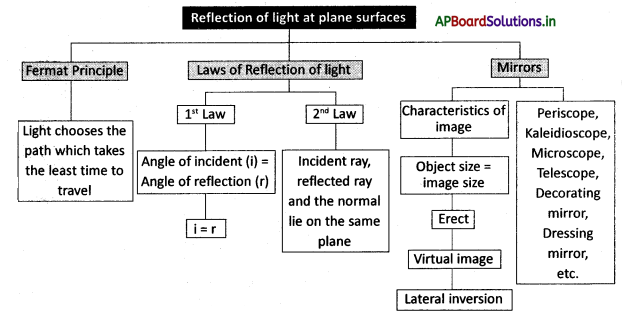# AP Board 8th Class Physical Science Notes Chapter 10 Reflection of Light at Plane Surfaces

Students can go through AP State Board 8th Class Physical Science Notes Chapter 10 Reflection of Light at Plane Surfaces to understand and remember the concept easily.

## AP State Board Syllabus 8th Class Physical Science Notes Chapter 10 Reflection of Light at Plane Surfaces

→ If In the reflection of the light angle of the incident (i), angle of reflection (r) are always equal.

→ Incident ray reflected ray and normal lie in the same plane. This plane is called the plane of reflection.

→ The image formed by the plane mirror is virtually erect and the same size as the object.

→ The object distance and image distance are the same in the case of the plane mirrors.

→ The image of an object in-plane mirror appears to be smaller when compared to its object because the angle of vision made by the image is smaller than that of the angle of vision made by the object.

→ In the plane mirror, the image is laterally inverted.

→ Fermat Principle: Light selects th€’path which takes the least time to travel.

→ Reflection: The bouncing back of the light is called reflection.

→ Incident ray: The ray which is incident on a surface is catted incident ray

→ Related ray The rd which ¡s reflected from the surface is called reflected ray

→Normal: The line perpendicular tå the surface is catted normal→ The angle of incidence: The angÍe between the incident ray and normal is called angle (∠i) of incidence

→ The angle of reflection: The angle between normal and reflected ray is called angle (∠r) of reflection

→ Plane of reflection: The plane in which the incident ray, reflected ray, and a normal lie is called the plane of reflection.

→ Lateral inversion: The image of an object reverses its direction on reflection is a lateral inversion (This means that objects that are on the right side, appear on the left side in the mirror.)

→ Object distance (u): The distance between object and mirror is &illed object distance.

→ Image distance (v): The distance between image and mirror is called image distance.

→ Virtual image: Virtual image ¡s the image formed due to diverging of light rays.
OR
The image formed due to the extension of rays in the backward direction.

→ Real image: The image formed due to the converging of light rays is real.→ Plane mirror: A plane mirror is that which has a planar reflective surface.

→ Ray diagram: A diagram showing the paths of selected rays through an optical system.→ Pierre de Fermat (17 August 1601 -12 January 1665):

• A French Lawyer at the Parliament of Toulouse, France, and an amateur mathematician.
• He made notable contributions to analytical geometry, probability, and optics.
• He proposed his principle on the light which states that light selects the path which takes the least time to travel. This principle was famous as the Fermat principle.